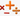## 加减乘除产生的表达式计数

Sun, 27th October 2019Edit on Githubalgorithmanalytic combination

2008年5月KeyTo9_Fans等在百度数学吧讨论由算24问题派生出来的计数问题，就是将n个不同的数字通过加减乘除运算（可以改变数字顺序和添加括号）连接起来 可以产生多少个不等价的表达式。其中比如(a+b)+c+d和a+(b+c)+d等价，因为可以通过我们熟悉的交换律分配率或结合律等相互转化。

mathe和KeyTo9_Fans共同出手并相互验证，最后mathe抢先得出n=100时，总共有 449917517701763281317016679046006077977987086161265203561131799463636866125482192454546567903120426734284631438483334078738257721979009093443435909627076270897944886935144957288232557564081388424946360387439835357455680511571869059290 个不等价的表达式。

# 详细内容

mathe给出了如下的算法的思路

$a-(b\times c-(d+e))$

$a-b\times c+d+e$

$a+d+e-b\times c$
$-b\times c+a+d+e$

$(b\times c, a, d, e)$

$\frac{\frac{a}{b+c}}{d\times e}$ 同样，我们可以把它改写成 $\frac{a}{(b+c)\times d\times e}$ 类似前面，我们把这个表达式看成分组
$(a, b+c, d, e)$

$\frac{a}{(b-c)}+d\times e$ 这个表达式同
$d\times e-\frac{a}{c-b}$

$x_1x_2..x_t$$y_1y_2...y_t$

2018年8月Zhujun Zhang使用解析组合得出更好的算法，将结果更新到了300项，比如第三百项是

72190028147018889750335485131328303931152274582550236089525162409795374552101273176794454983868785962708688202710181198684352866146727661739564511680864977514566139496879769349359289549566028996044476516485895050609304963244258584965660688879810583890530231617438520357138548072805455108020217067359180748422323864413955769641649473209885524803873657901023082852855074512602102118234803504483350613423240988997188280088539348565629602280338815644982671693200891519489372327809082142923858939068708053619624995070063973108938476056358131172343896608014407677212410031033341157688603637733542123642865148016593343643219416232187380709150093319011151323845637138861981383252936745634980311080707083425397147421602918476666397675833977564301679029137943021579679971179004749690773848817257553051528298367371360988489078773939477280125867271746491441210

Github